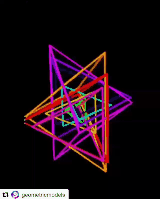top of page
Search

# Sacred geometry behind time numbers

Updated: Apr 11, 2021

2 Kathara grids joined together form the Cubistic matrix Diagram was removed.

Time numbers are based on the numbers 12, 24 and 60 but overall they can be turned into base 12 forming:

• 12:12=Kathara grid:Kathara grid

As we know the Kathara grid has 28 paths so 12:12 encodes

• Kathara grid:Kathara grid=28:28

Whats interesting about this geometry is that 28:28=56 and 56 forms the basic structure of the 64 tetrahedron grid also the Kathara grid is encoded in the star tetrahedron and 12:12=Star tetrahedron:Star tetrahedron and from this we can place a star tetrahedron inside the other and form the fruit of life which encodes Metatrons cube/64 tetrahedron grid and this forms a fractaling star tetrahedron which forms the Sri Yantra which corresponds to E8 and the I Ching which are both forms of the 64 tetrahedron grid.The star tetrahedron contained within a star tetrahedron structure also encodes vibrational dimensions as we know and this structure corresponds to time numbers which already correspond to vibrational dimensions because time numbers are basically 2 multidimensional polygons joined together which both correspond to tetractyses(Which correspond to tetrahedron grids and dimensions) and they both form a 3D multidimensional polygon which corresponds to the cosmic tree of life which is formed out of the powers of 3 so it is base 3 and it is the vibrational dimensions.

The Hebrew alphabet is base 3 and when its in base 3 it forms the 231 gates and it also forms a 3 by 3 cube so it forms the cubistic matrix and cosmic tree of life.